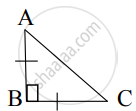# From given figure, In ∆ABC, AB ⊥ BC, AB = BC then m∠A = ? - Geometry

Sum

From given figure, In ∆ABC, AB ⊥ BC, AB = BC then m∠A = ?#### Solution

AB = BC     ......[Given]

∴ ∠A = ∠C   ......[Isosceles triangle theorem]

Let ∠A = ∠C = x

In ∆ABC,

∠A + ∠B + ∠C = 180°    ...[Sum of the measures of the angles of a triangle is 180°]

∴ x + 90° + x = 180°

∴ 2x = 90°

∴ x = (90°)/2

∴ x = 45°

∴ m∠A = 45°

Concept: Application of Pythagoras Theorem in Acute Angle and Obtuse Angle
Is there an error in this question or solution?
Chapter 2: Pythagoras Theorem - Q.1 (B)

Share